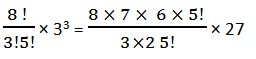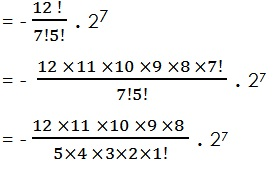Study Materials

# NCERT Solutions for Class 11th Mathematics

Page 2 of 3

## Chapter 8. Binomial Theorem

### Exercise 8.2

Exercise 8.2

Find the coefficient of

Q1.  x5 in (x + 3)8

Solution:

(x + 3)8

General term nCr an-r br

n = 8, then

8Cr x8-r 3r x5 = x8-r

5 = 8-r

∴ r = 8-5 = 3

8C3 x8-3 33 = 8C3 x5 33

Coefficient in x5 = 8C3 33= 1512

Q2.  a5b7 in (a-2b)12

Solution:

(a-2b)12

General Term Tr+1 = nCr an-r br

12Cr a12-r br

12 – r = 5  r = 12 – 5 = 7

a5b7 is 8 term

T8 = 12C7 a5 b7

= 12C7 a5 (-2b)7= - 72 × 11 × 27

= - 72 × 11 × 128 = - 101376

Write the general term in the expansion of

Q3. (x2 - y)6

Solution:

General term of (x2 - y)6

n = 6, a = x2, b = (-y)

General Term Tr +1nCan-r br

=  6Cr (x2)6-r (-y)r

Page 2 of 3

Chapter Contents: